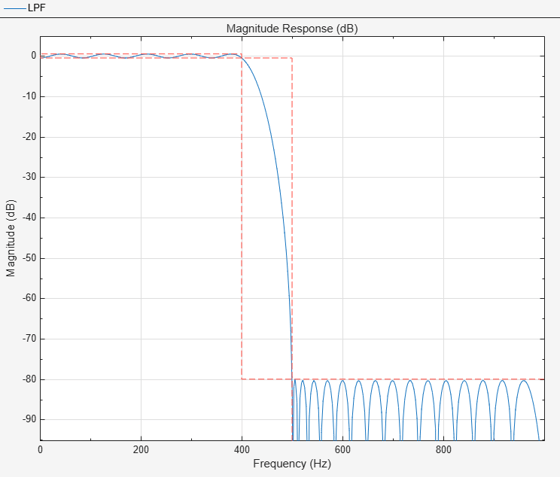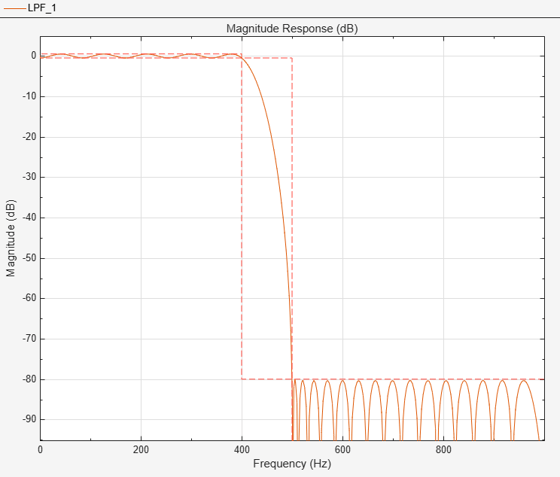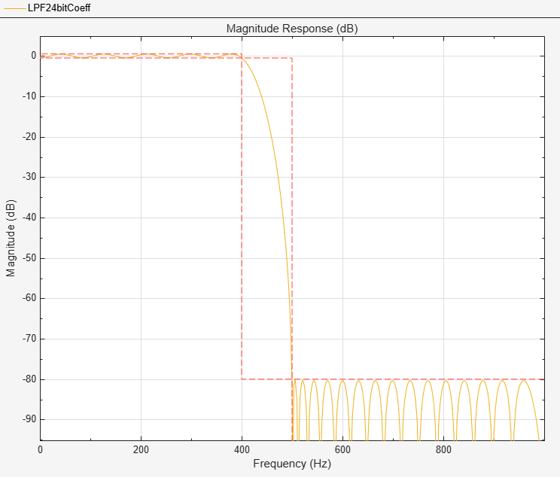Design filters for use with fixed-point input. The example analyzes the effect of coefficient quantization on filter design. You must have the Fixed-Point Designer™ software to run this example.

### Introduction

Fixed-point filters are commonly used in digital signal processors where data storage and power consumption are key limiting factors. With the constraints you specify, DSP System Toolbox™ software allows you to design efficient fixed-point filters. The filter for this example is a lowpass equiripple FIR filter. Design the filter first for floating-point input to obtain a baseline. You can use this baseline for comparison with the fixed-point filter.

### FIR Filter Design

The lowpass FIR filter has the following specifications:

• Sample rate: 2000 Hz

• Center frequency: 450 Hz

• Transition width: 100 Hz

• Equiripple design

• Maximum 1 dB of ripple in the passband

• Minimum 80 dB of attenuation in the stopband

```samplingFrequency = 2000; centerFrequency = 450; transitionWidth = 100; passbandRipple = 1; stopbandAttenuation = 80; designSpec = fdesign.lowpass('Fp,Fst,Ap,Ast',... centerFrequency-transitionWidth/2, ... centerFrequency+transitionWidth/2, ... passbandRipple,stopbandAttenuation, ... samplingFrequency); LPF = design(designSpec,'equiripple',... 'SystemObject',true)```
```LPF = dsp.FIRFilter with properties: Structure: 'Direct form' NumeratorSource: 'Property' Numerator: [-0.0013 -0.0055 -0.0112 -0.0125 -0.0048 0.0062 0.0081 -0.0022 -0.0104 -0.0027 0.0115 0.0093 -0.0098 -0.0171 0.0039 0.0242 0.0073 -0.0286 -0.0247 0.0268 0.0498 -0.0134 -0.0887 -0.0298 0.1895 0.3983 0.3983 0.1895 -0.0298 ... ] InitialConditions: 0 Show all properties ```

View the baseline frequency response. The dotted red lines show the design specifications used to create the filter.

`fvtool(LPF)`### Full-Precision Fixed-Point Operation

The fixed-point properties of the filter are contained in the `Fixed-point properties` section in the display of the object. By default, the filter uses full-precision arithmetic to deal with fixed-point inputs. With full-precision arithmetic, the filter uses as many bits for the product, accumulator, and output as needed to prevent any overflow or rounding. If you do not want to use full-precision arithmetic, you can set the `FullPrecisionOverride` property to `false` and then set the product, accumulator, and output data types independently.

```rng default inputWordLength = 16; fixedPointInput = fi(randn(100,1),true,inputWordLength); floatingPointInput = double(fixedPointInput); floatingPointOutput = LPF(floatingPointInput); release(LPF) fullPrecisionOutput = LPF(fixedPointInput); norm(floatingPointOutput-double(fullPrecisionOutput),'inf')```
```ans = 6.8994e-05 ```

The result of full-precision fixed-point filtering comes very close to floating point, but the results are not exact. The reason for this is coefficient quantization. In the fixed-point filter, the `CoefficientsDataType` property has the same word length (16) for the coefficients and the input. The frequency response of the filter in full-precision mode shows this more clearly. The `measure` function shows that the minimum stopband attenuation of this filter with quantized coefficients is 76.6913 dB, less than the 80 dB specified for the floating-point filter.

`LPF.CoefficientsDataType`
```ans = 'Same word length as input' ```
`fvtool(LPF)``measure(LPF)`
```ans = Sample Rate : 2 kHz Passband Edge : 400 Hz 3-dB Point : 416.2891 Hz 6-dB Point : 428.1081 Hz Stopband Edge : 500 Hz Passband Ripple : 0.96325 dB Stopband Atten. : 76.6913 dB Transition Width : 100 Hz ```

The filter was last used with fixed-point input and is still in a locked state. For that reason, `fvtool` displays the fixed-point frequency response. The dash-dot response is that of the reference floating-point filter, and the solid plot is the response of the filter that was used with fixed-point input. The desired frequency response cannot be matched because the coefficient word length has been restricted to 16 bits. This accounts for the difference between the floating-point and fixed-point designs. Increasing the number of bits allowed for the coefficient word length makes the quantization error smaller and enables you to match the design requirement for 80 dB of stopband attenuation. Use a coefficient word length of 24 bits to achieve an attenuation of 80.1275 dB.

```LPF24bitCoeff = design(designSpec,'equiripple',... 'SystemObject',true); LPF24bitCoeff.CoefficientsDataType = 'Custom'; coeffNumerictype = numerictype(fi(LPF24bitCoeff.Numerator,true,24)); LPF24bitCoeff.CustomCoefficientsDataType = numerictype(true,... coeffNumerictype.WordLength,coeffNumerictype.FractionLength); fullPrecisionOutput32bitCoeff = LPF24bitCoeff(fixedPointInput); norm(floatingPointOutput-double(fullPrecisionOutput32bitCoeff),'inf')```
```ans = 4.1077e-07 ```
`fvtool(LPF24bitCoeff)``measure(LPF24bitCoeff)`
```ans = Sample Rate : 2 kHz Passband Edge : 400 Hz 3-dB Point : 416.2901 Hz 6-dB Point : 428.1091 Hz Stopband Edge : 500 Hz Passband Ripple : 0.96329 dB Stopband Atten. : 80.1275 dB Transition Width : 100 Hz ```

### Design Parameters and Coefficient Quantization

In many fixed-point design applications, the coefficient word length is not flexible. For example, supposed you are restricted to work with 14 bits. In such cases, the requested minimum stopband attenuation of 80 dB cannot be reached. A filter with 14-bit coefficient quantization can achieve a minimum attenuation of only 67.2987 dB.

```LPF14bitCoeff = design(designSpec,'equiripple',... 'SystemObject',true); coeffNumerictype = numerictype(fi(LPF14bitCoeff.Numerator,true,14)); LPF14bitCoeff.CoefficientsDataType = 'Custom'; LPF14bitCoeff.CustomCoefficientsDataType = numerictype(true, ... coeffNumerictype.WordLength,coeffNumerictype.FractionLength); measure(LPF14bitCoeff,'Arithmetic','fixed')```
```ans = Sample Rate : 2 kHz Passband Edge : 400 Hz 3-dB Point : 416.2939 Hz 6-dB Point : 428.1081 Hz Stopband Edge : 500 Hz Passband Ripple : 0.96405 dB Stopband Atten. : 67.2987 dB Transition Width : 100 Hz ```

For FIR filters in general, each bit of coefficient word length provides approximately 5 dB of stopband attenuation. Accordingly, if your filter's coefficients are always quantized to 14 bits, you can expect the minimum stopband attenuation to be only around 70 dB. In such cases, it is more practical to design the filter with stopband attenuation less than 70 dB. Relaxing this requirement results in a design of lower order.

```designSpec.Astop = 60; LPF60dBStopband = design(designSpec,'equiripple',... 'SystemObject',true); LPF60dBStopband.CoefficientsDataType = 'Custom'; coeffNumerictype = numerictype(fi(LPF60dBStopband.Numerator,true,14)); LPF60dBStopband.CustomCoefficientsDataType = numerictype(true, ... coeffNumerictype.WordLength,coeffNumerictype.FractionLength); measure(LPF60dBStopband,'Arithmetic','fixed')```
```ans = Sample Rate : 2 kHz Passband Edge : 400 Hz 3-dB Point : 419.3391 Hz 6-dB Point : 432.9718 Hz Stopband Edge : 500 Hz Passband Ripple : 0.92801 dB Stopband Atten. : 59.1829 dB Transition Width : 100 Hz ```
`order(LPF14bitCoeff)`
```ans = 51 ```
`order(LPF60dBStopband)`
```ans = 42 ```

The filter order decreases from 51 to 42, implying that fewer taps are required to implement the new FIR filter. If you still want a high minimum stopband attenuation without compromising on the number of bits for coefficients, you must relax the other filter design constraint: the transition width. Increasing the transition width might enable you to get higher attenuation with the same coefficient word length. However, it is almost impossible to achieve more than 5 dB per bit of coefficient word length, even after relaxing the transition width.

```designSpec.Astop = 80; transitionWidth = 200; designSpec.Fpass = centerFrequency-transitionWidth/2; designSpec.Fstop = centerFrequency+transitionWidth/2; LPF300TransitionWidth = design(designSpec,'equiripple',... 'SystemObject',true); LPF300TransitionWidth.CoefficientsDataType = 'Custom'; coeffNumerictype = numerictype(fi(LPF300TransitionWidth.Numerator,... true, 14)); LPF300TransitionWidth.CustomCoefficientsDataType = numerictype(true,... coeffNumerictype.WordLength,coeffNumerictype.FractionLength); measure(LPF300TransitionWidth,'Arithmetic','fixed')```
```ans = Sample Rate : 2 kHz Passband Edge : 350 Hz 3-dB Point : 385.4095 Hz 6-dB Point : 408.6465 Hz Stopband Edge : 550 Hz Passband Ripple : 0.74045 dB Stopband Atten. : 74.439 dB Transition Width : 200 Hz ```

As you can see, increasing the transition width to 200 Hz allows 74.439 dB of stopband attenuation with 14-bit coefficients, compared to the 67.2987 dB attained when the transition width was set to 100 Hz. An added benefit of increasing the transition width is that the filter order also decreases, in this case from 51 to 27.

`order(LPF300TransitionWidth)`
```ans = 27 ```